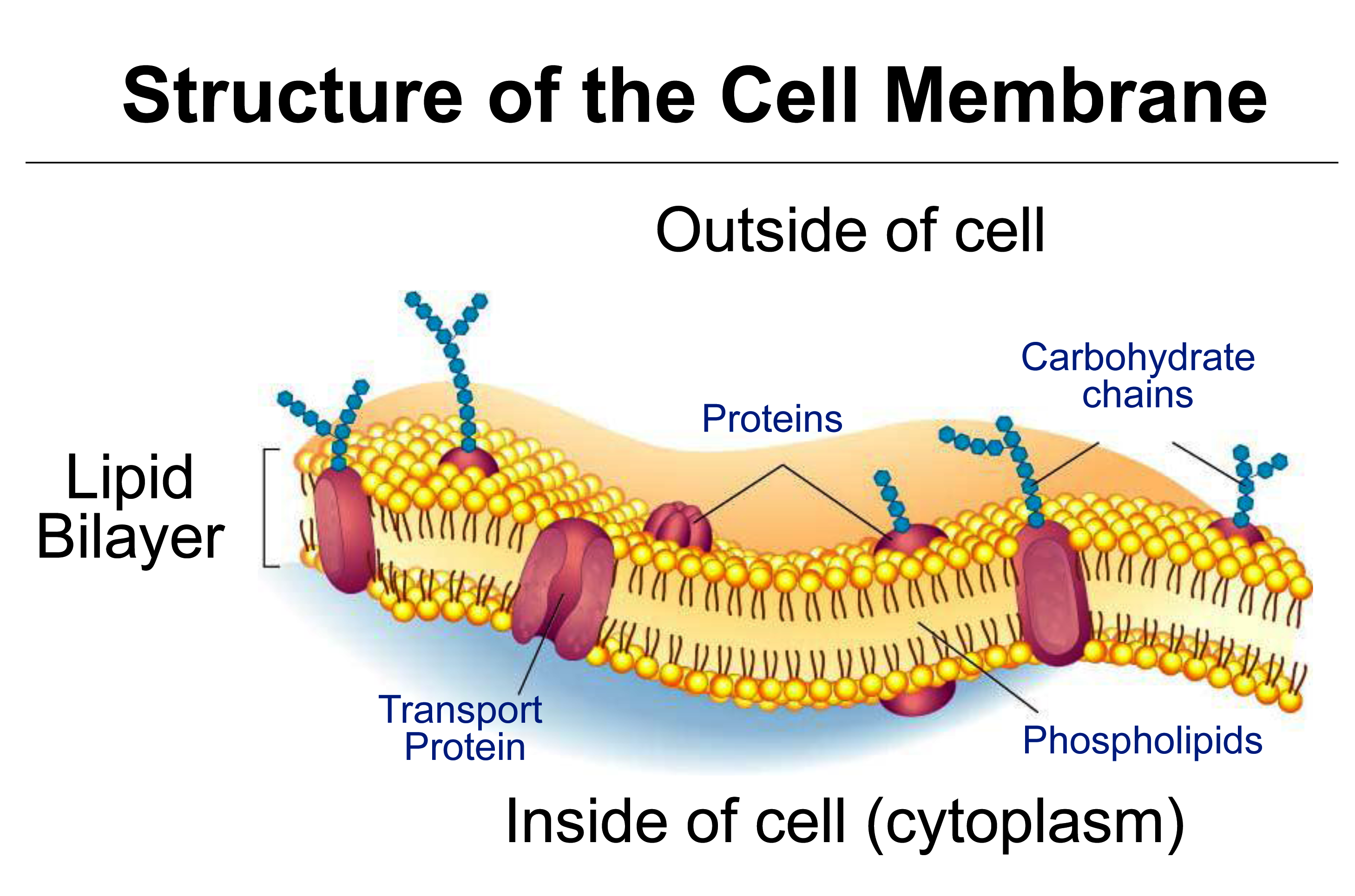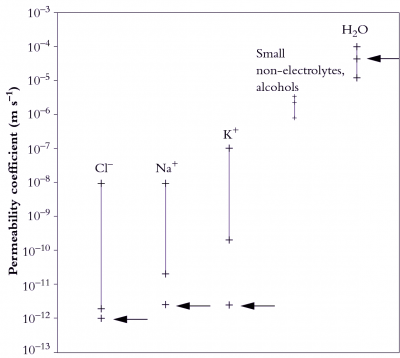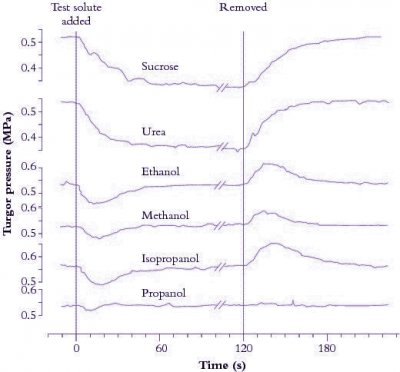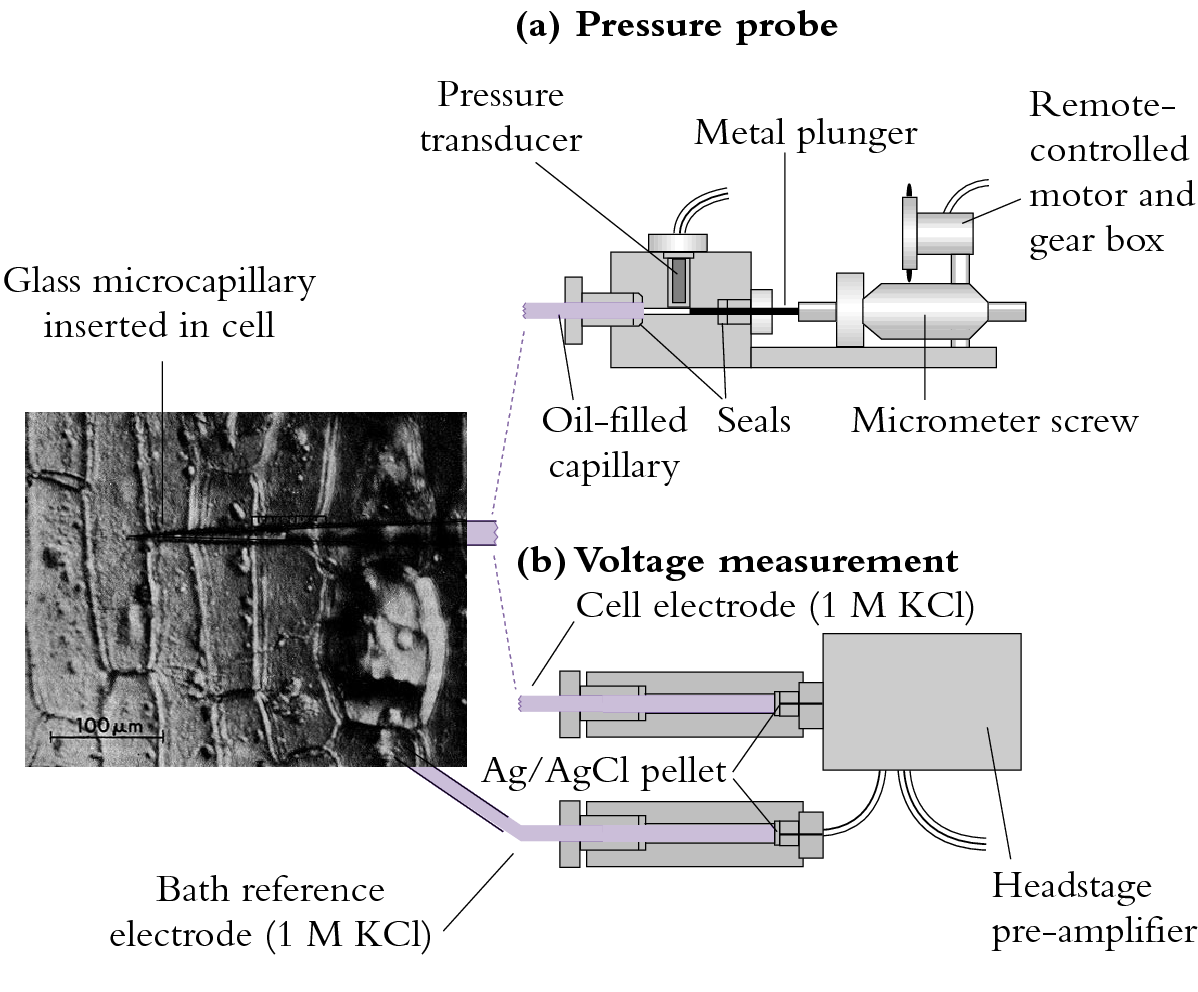# 3.6.1 - Diffusion and permeability

This section covers diffusion of molecules, and permeability of cell membranes, essential to the process of osmosis. Cell membranes are bilayers of phospholipids in which transport proteins are embedded (Figure 3.65).

## 3.0-Ch-Fig-3.65.pngFigure 3.65 Diagram of a cell membrane with transport proteins embedded in the phospholiped bilayers. (Image courtesy M. Hrmova)

A plant membrane is often described as semi-permeable, meaning permeable to water but not biological solutes. However the membrane is not 100% permeable to water, as water can enter cells only by being transported through aquaporins, neither is it 100% impermeable to solutes, as solutes can slowly permeate the membrane particularly through specific transport proteins.

Unrestricted movement of water relative to solutes is the basis of osmosis, and in plants the generation of turgor pressure, $P$. The principles of diffusion and selectivity, which are used to describe differential rates of molecular movement, provide a physical rationale for osmosis.

### (a) Diffusion

In a homogeneous medium, net movement of molecules down their concentration gradient is described by Fick’s First Law of diffusion. The molecule and medium may be a solute in water, a gas in air or a molecule within the lipid bilayer. Fick’s Law holds when the medium is homogeneous in all respects except for the concentration of the molecule. If there was an electric ﬁeld or a pressure gradient then Fick’s Law may not apply. Considering the case of a solute in water, say, sugar, Fick’s Law states that net movement of this solute, also called the net flux $J_s$, is proportional to the concentration gradient of the solute $\Delta C_s / \Delta x$:

$J_s = -D_s \frac{\Delta C_s}{\Delta x} \tag{12}$

The diffusion coefﬁcient ($D_s$) is a constant of proportionality between flux, $J_s$, and concentration gradient (mol m-4), where solute concentration ($C_s$, mol m-3) varies over a distance ($\Delta x$, m). Flux is measured as moles of solute crossing a unit area per unit time (mol m–2 s–1), so $D_s$ has the units m2 s–1. $D_s$ has a unique value for a particular solute in water which would be quite different from $D_s$ for the same solute in another medium, for example the oily interior of a lipid membrane.

Across a membrane, the movement of a molecule from one solution to another can be described by Fick’s Law applied to each phase (solution 1–membrane–solution 2). However, flux across the membrane also depends on the ability of the molecule to cross boundaries (i.e. to partition) from solution into the hydrophobic membrane and then from the membrane back into solution. Another difﬁculty is that the thickness of membranes is relatively undeﬁned and we need to know this for Fick’s equation above ($\Delta x$). The two solutions might differ in pressure and voltage and these can change steeply across a membrane; however, if for simplicity we consider a neutral solute at low concentration, these factors are not relevant (see below for charged molecules). A practical quantitative description of the flux of neutral molecules across membranes uses an expression intuitively related to Fick’s Law stating that flux across a membrane ($J_s$) of a neutral molecule is proportional to the difference in concentration $\Delta C_s$:

$J_s = P_s \Delta C_s \tag{13}$

## 3.0-Ch-Fig-3.66.pngFigure 3.66. The range of permeability coefficients for various ions, solutes and water in plant membranes (vertical bars) and artificial phospholipids (arrows). Note that the permeability of ions as they cross plant membranes is higher than through the artificial lipid bilayer.

The constant of proportionality in this case is the permeability coefﬁcient ($P_s$), expressed in m s–1. When $P_s$ is large, solutes will diffuse rapidly across a membrane under a given concentration gradient. $P_s$ embodies several factors: partitioning between solution and membrane, membrane thickness and diffusion coefﬁcient of the solute in the membrane which may be largely depend on specific transporters. It can be used to compare different membranes and to compare treatments that might alter the ability of a solute to move across the membrane.

Note that Equation 13 assumes that the concentration gradient is only across the membrane, and that when the permeability coefficient is measured, concentration gradients leading to diffusion in solutions adjacent to the membrane will not be signiﬁcant. If the two solutions are stirred rapidly then this will help to justify this assumption. However, there is always an unstirred layer adjacent to the membrane through which diffusion occurs, and for molecules that can permeate the membrane very rapidly the unstirred layer can be a problem for the correct measurement of permeability.

### (b) Permeabilities

Solute movement across membranes can be across the lipid phase of the membrance, depending on size, charge and polarity, and it can be assisted by transport proteins embedded in the membrane.

The range of permeability coefficients for various ions, solutes and water in plant membranes is shown in Figure 3.66, along with the permeability of artificial phospholipid bilayers. The permeability of ions as they cross membranes is higher than that through the artificial lipid bilayer, especially for potassium, indicating the presence of specialised permeation mechanisms, ion transporters, in plant membranes. Water permeabilities are high in both plant and artificial membranes but can range over an order of magnitude in plant membranes. This variability may be partially accounted for by the activity of aquaporins.

A comparison of artiﬁcial lipid membranes with biological membranes supports this notion because it shows that many molecules and ions permeate biological membranes much faster than would be predicted on the basis of oil solubility and size (Figure 3.66). For these solutes there are transport proteins in biological membranes that increase solute permeability.

### (c) Reflection coefficient - water versus solute permeability

Plant membranes are ideally semipermeable, that is, water permeability is much larger than solute permeability.

The degree of semi-permeability that a membrane shows for a particular solute is measured as the reflection coefﬁcient, $\sigma$:

$\sigma = 1 - \frac{\text{Solute Permeability}}{\text{Water Permeability}} \tag{15}$

## 3.0-Ch-Fig-3.67.jpgFigure 3.67. Turgor pressure ($P$) in a Tradescantia virginiana epidermal cell as a function of time after the external osmotic pressure was changed with different test solutes. Measurements were made with a pressure probe. (Tyerman and Steudle 1982)

If a plant cell or an epidermal strip is bathed in solution, the reflection coefficient for a particular solute can also be considered as the ratio of the effective osmotic pressure versus the actual osmotic pressure in the bathing solution.

The reflection coefﬁcient usually ranges between zero and one, being zero for molecules with properties similar to water, like methanol, to one for large non-polar molecules like sucrose.

Figure 3.67 shows turgor pressure ($P$) in a Tradescantia virginiana epidermal cell as a function of time after the external osmotic pressure was changed with different test solutes. The initial decrease in $P$ is due to water flow out of the cell and is larger for solutes with a reflection coefficient near one (sucrose and urea). Propanol induces no drop in $P$, indicating that its reflection coefficient is zero. Subsequent increase in $P$ is due to penetration of particular solutes such as alcohol across the cell membrane. Water flows osmotically with the solute thereby increasing $P$ to its original value. Removing solutes reverses osmotic effects. That is, a decrease in $P$ follows the initial inflow of water as solutes (e.g. alcohols) diffuse out of cells.

The pressure probe apparatus is illustrated in Figure 3.68(a).

## 3.0-Ch-Fig-3.68.pngFigure 3.68 (a) A miniaturised pressure probe. An oil-filled capillary of about 1 µm diameter is inserted into a cell whose turgor pressure ($P$) is transmitted through the oil to a miniature pressure transducer. The voltage output of the transducer is proportional to $P$. A metal plunger acting as a piston can be used via remote control to vary cell $P$.

Using the pressure probe to measure turgor pressure, $P$, the membrane is found to be ideally semipermeable for sucrose ($\sigma = 1$); that is, the membrane almost totally ‘reflects’ sucrose. Over long periods, sucrose is taken up slowly but permeability relative to water is negligible. In this case, the change in $P$ would be equivalent to the change in $\pi$. If $\sigma$ is near zero, then water and the solute (say, propanol) are equivalent in terms of permeability. No change in $P$ can be generated across a cell wall if $\sigma$ is zero.